Next: Example of calculation Up: Cookbook Formulae for Previous: Dead times

## Examples of calculation of time efficiency ratios

• A) PSW scan: The time needed for a position switch scan (8 subscans with integration time= 30s ON source or on OFF position atfrom the source, set subs 8, psw /t 30 /sym) is thus roughly:the efficiency ratio (integration time/observing time) is.

• B) WSW scan: The time needed for a wobbler switch scan (8 subscans with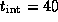s,
set subs 8, wsw 240 /t 40 /p 2) is: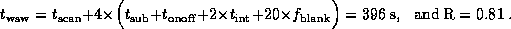• C) RASTER scan: The time needed for a `Raster' scan (i.e. several ONs for one OFF by using the OBS command `RASTER') can be estimated from the values of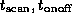, and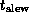given above. For a raster scan consisting of 15 ONs of 30 s and 8 OFFs of 45 s, such as:

RASTER 84 6 0 6/REF 3600 0/ON 2/T 30 45,

the calculation gives: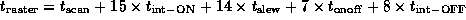,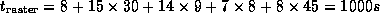and R= 0.78.

Note that the measured average time for such a raster scan was actually 1050 s and R= 0.74.

• D)For a 3-hour observation on one object, one must add 3 pointings and one focussing, and at least 10 calibrations: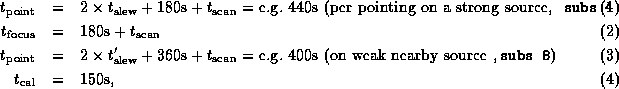i.e., or 2500s (0.7h), to which you may add your own reaction time, typing time, etc...

In total, in this typical PSW mode, you can expect to observe in 3 hours (10800 s) about (10800-2500)/scans (total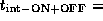7000s (2h)). The observing `efficiency' is thus only.

In the Wobbling mode, these numbers would drop to 21 scans,s and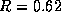. (You could increase them somewhat by setting set subs 12).

• E) For a `complete' 8-hour long PSW or WSW observation on the same object, with one single tuning (plus one retuning) per receiver, you have (3 receivers operating simultaneously):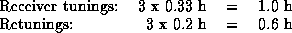The following preparation costs about 0.4 hours:

First slew to the source: 5 min
Skydip: 5 min
Observation of a Line Calibrator: 15 min (with slew)

Observations: 6 h (including 3.7 to 3.9 h of integration ON+OFF source, see example C above).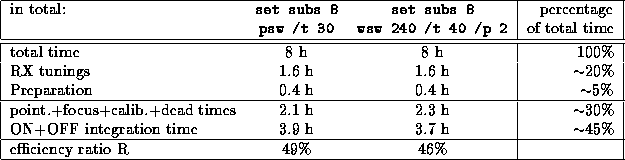Next: Example of calculation Up: Cookbook Formulae for Previous: Dead times

Robert Lucas
Thu Mar 9 12:14:01 MET 1995